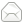# It may be a fun game...But What Do I Have to Improve this code ?? (Sorry, I'm not a American...)

# Jumping Ball

game_over_text = "GAME OVER"

wall = '#'*32
guide = '#' + ' '*30 + '#'

rock = 'A'
ball_x = 5
ball_y = 9
rock1_x = 30
rock2_x = 42
score = 0
jump = False
jump_count = 0
end = False

def clear():
os.system('cls' if os.name == 'ct' else 'clear')

def set():
print(wall)
print('#'+' score: ',end='')
str_score = str(score)
score_len = len(str_score)
print(  str_score + ' ' * ( 30 - ( 8 + score_len ) ) + '#' )
for yy in range(2,10):
for xx in range(0, len(guide)): #

if yy == ball_y and xx == ball_x :
print(ball, end='')

elif ( xx == rock1_x or xx == rock2_x ) and yy == 9 :
print(rock, end='')

else :
print(guide[xx],end='')
print()
print(wall)

def __init__(self, var):
self.put = var

def run(self):
global score, rock1_x, rock2_x, ball_x, ball_y, jump, jump_count, end
if self.put :
while 1:
input('>>>')
jump = True

else :
while 1:
set()

if jump :
if jump_count <= 5:
jump_count += 1
ball_y = 4

else :
jump_count = 0
jump = False
ball_y = 9

elif rock1_x == ball_x or rock1_x == ball_x:
end = True
print(game_over_text)
print('\nYou scored %d points' % score)
break

if rock1_x == 1:
rock1_x = 30
score += 1

if rock2_x == 1:
rock2_x = 30
score += 1

rock1_x -= 1
rock2_x -= 1

time.sleep(0.1)
clear()

a = SYSTEM(True)
b = SYSTEM(False)

b.start()
a.start()

answered Apr 24 by (350 points)
lol i want to know how the game works
commented Apr 24 by (72,620 points)
You have to jump over obstacles denoted by 'A' by pressing ENTER at the right time. The more you dodge the more score you get.
+1 vote
answered Apr 24 by (72,620 points)

Here are some gameplay ideas

• randomize the distance between the two rocks.
• randomize how many rocks you have (1, 2, 3, etc)
• with time, increase difficulty (more rocks, higher speed)
• add tree branches (or something in the air) that you're not supposed to hit. So jump over the rocks, but don't hit your head in the tree branches.
Here are some code ideas
• use classes for encapsulation
• move from "interactive console game" to Pygame or Unity (C#).and jump again and again